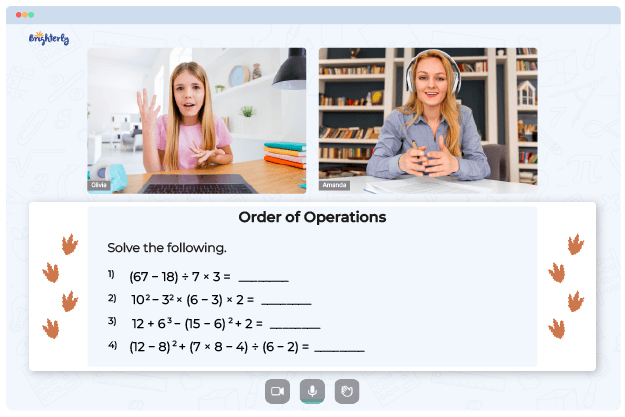# Order of Operations with Exponents Worksheets

Order of operations with exponents is a hard topic, so kids often need all the help they can get with this aspect of exponents. You can use the order of operations with exponents worksheets to help kids better understand the order of operations with exponents.

## Benefits of the order of operations with exponents worksheets

Here are some of the benefits of an order of operations worksheet with exponents:

### It helps kids understand the correct order to follow

Continuous practice with the order of operations with the exponents worksheet PDF will help kids learn the correct order of operations to perform them properly.

Math for Kids

Is Your Child Struggling With Math?
1:1 Online Math Tutoring### It reduces the amount of work the kids have to do

With the order of operations with exponents worksheet, kids can learn how to use exponents to eliminate smaller math operations like addition, subtraction, and multiplication so that they can quickly move on to the more complex parts of the math problems.

You can download the order of operations with exponents worksheet pdf online and print exponents and order of operations worksheets out for kids in your class to practice at home.### Order of Operations Worksheet with Exponents PDF

Order of Operations Worksheet with Exponents### Order of Operations with Exponents Worksheet PDF

Order of Operations with Exponents Worksheet### Order of Operations with Exponents Worksheet PDF

Order of Operations with Exponents Worksheet PDF### Exponents and Order of Operations Worksheets PDF

Exponents and Order of Operations Worksheets

Troubles with order of operations?• Does your child need extra support with understanding order of operations?
• Start lessons with an online tutor.

Is your child finding it hard to understand the fundamentals of order of operations? An online tutor could be of help.